# 前言

AI 作画从 18 年的 DeepDream噩梦中惊醒过来，在 2022 年 OpenAI 的 DALL·E 2达到惊人效果，见图：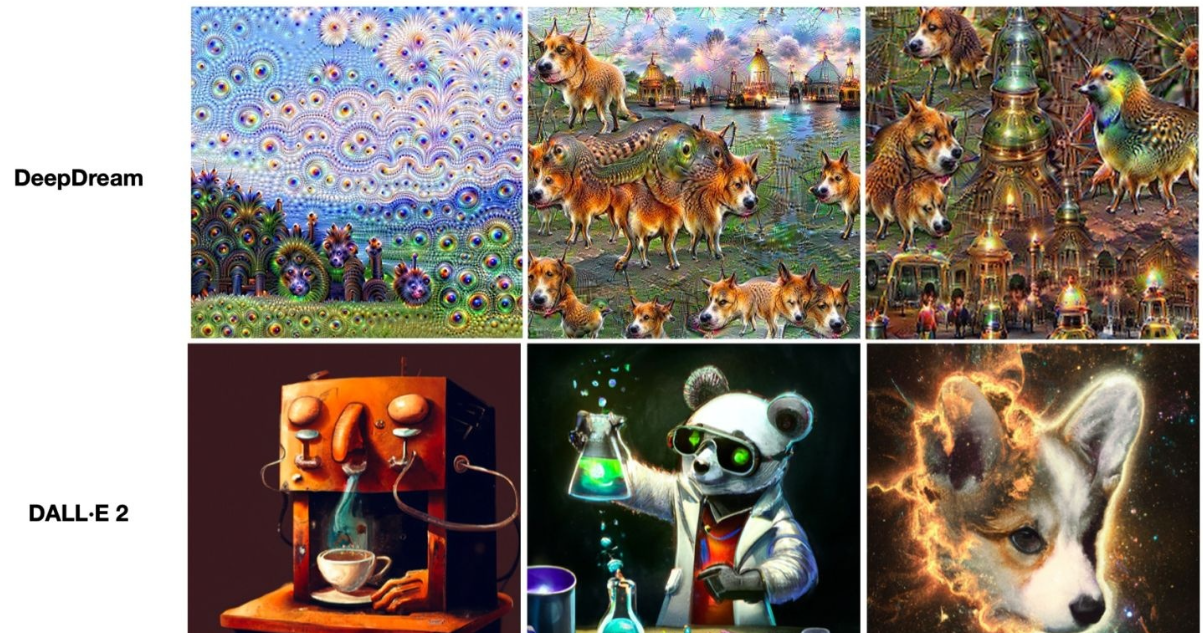AI + 艺术涉及到 Transformer、VAE、ELBO、Diffusion Model 等一系列跟数学相关的知识。Diffusion Models 跟 VAE 一样原理很复杂

# 一、常见生成模型

GAN 由一个生成器（generator）和判别器（discriminator）组成，generator 负责生成逼真数据以 “骗” 过 discriminator，而 discriminator 负责判断一个样本是真实的还是 “造” 出来的。GAN 的训练其实就是两个模型在相互学习，能不能不叫“对抗”，和谐一点。

VAE 同样希望训练一个生成模型 x=g(z)，这个模型能够将采样后的概率分布映射到训练集的概率分布，生成隐变量 z ，并且 z 是既含有数据信息又含有噪声，除了还原输入的样本数据以外，还可以用于生成新的数据。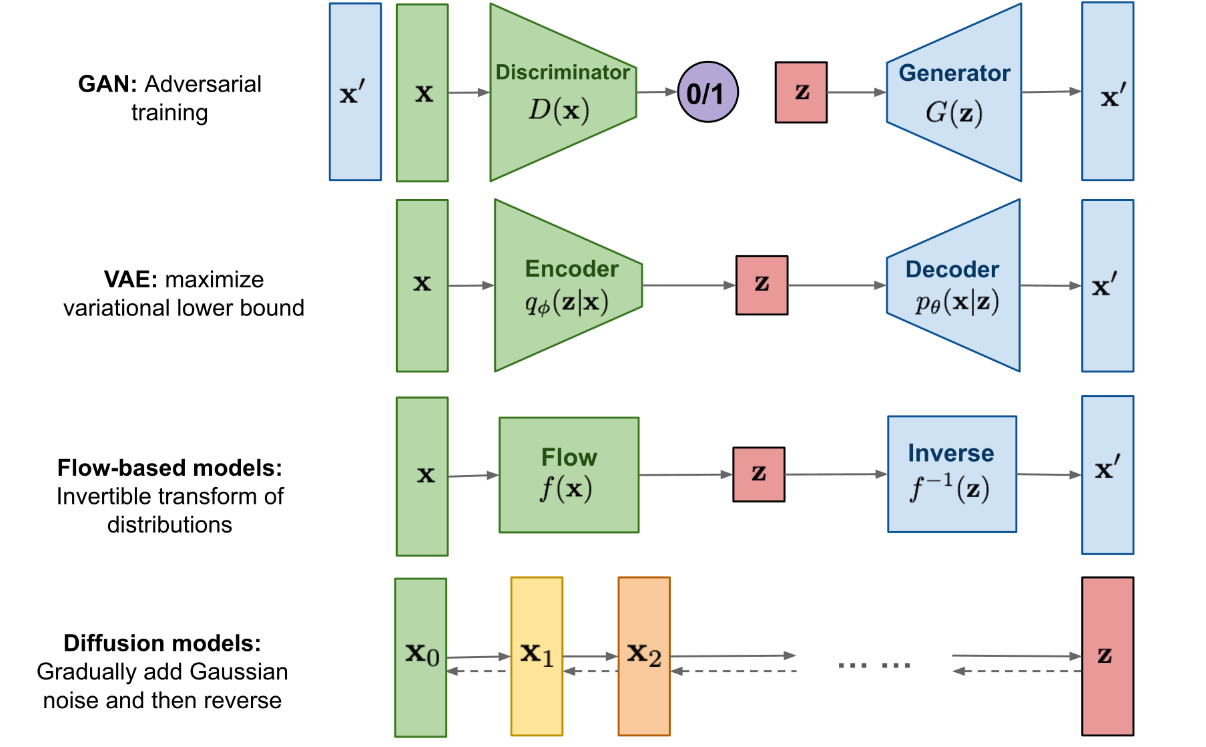Diffusion Models 的灵感来自non-equilibrium thermodynamics （非平衡热力学）。理论首先定义扩散步骤的马尔可夫链，以缓慢地将随机噪声添加到数据中，然后学习逆向扩散过程以从噪声中构造所需的数据样本。与 VAE 或流模型不同，扩散模型是通过固定过程学习，并且隐空间 z 具有比较高的维度。

# 二、直观理解Diffusion model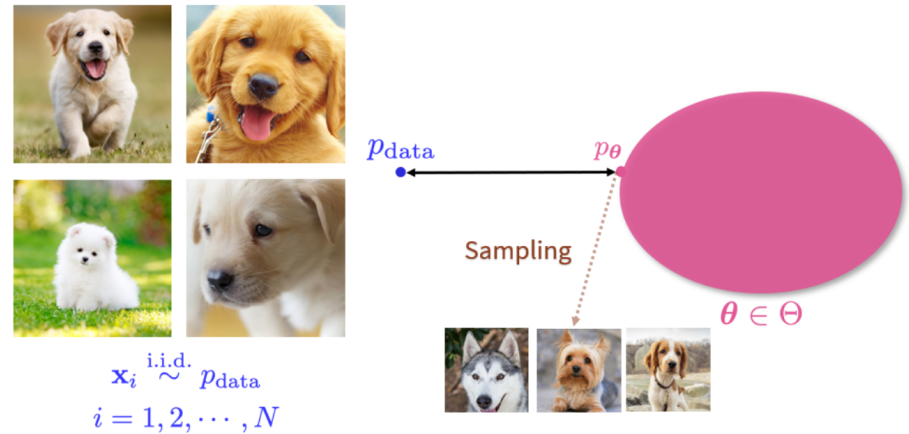Diffusion作用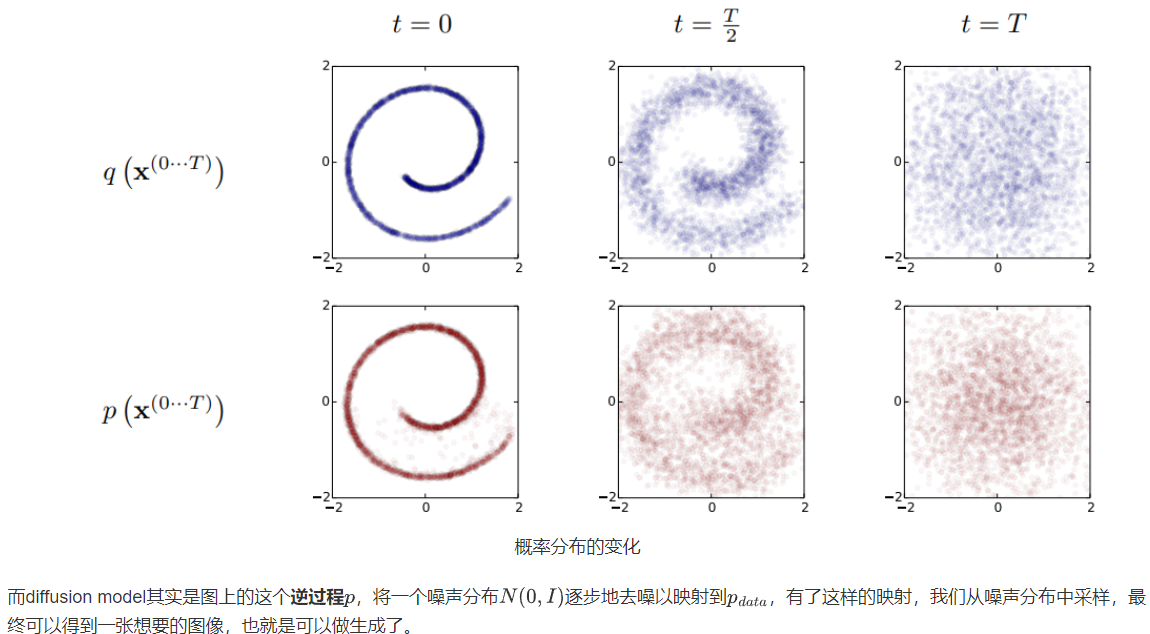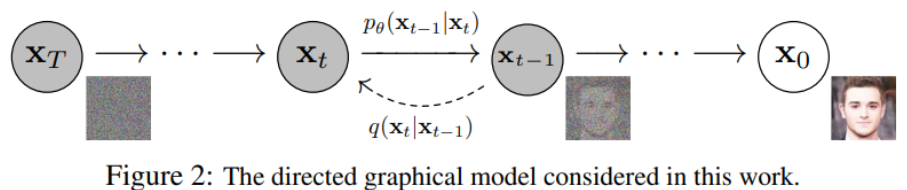# 三、形式化解析Diffusion model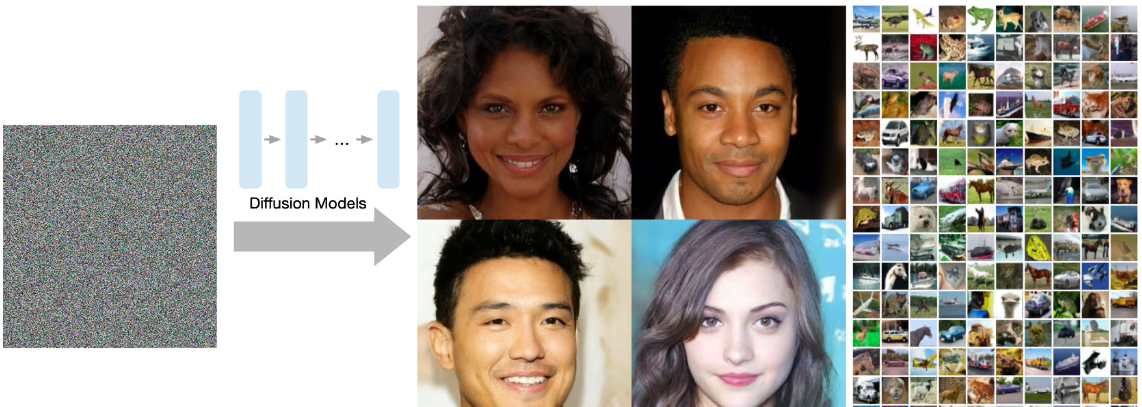Diffusion Models 分为正向的扩散过程和反向的逆扩散过程。下图为扩散过程，从 到最后的 就是一个马尔可夫链，表示状态空间中经过从一个状态到另一个状态的转换的随机过程。而下标则是 Diffusion Models 对应的图像扩散过程。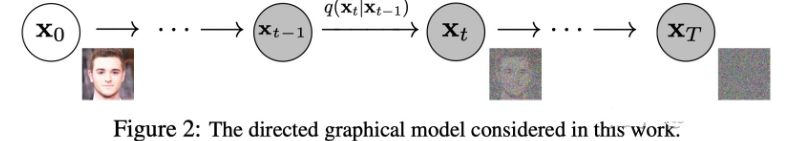Diffusion Models 跟 GAN 或者 VAE 的最大区别在于不是通过一个模型来进行生成的，而是基于马尔可夫链，通过学习噪声来生成数据。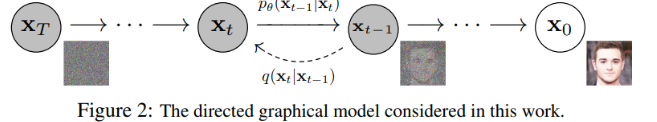# *四、详解 Diffusion Model（数学推导）

## 1.前向过程(扩散过程)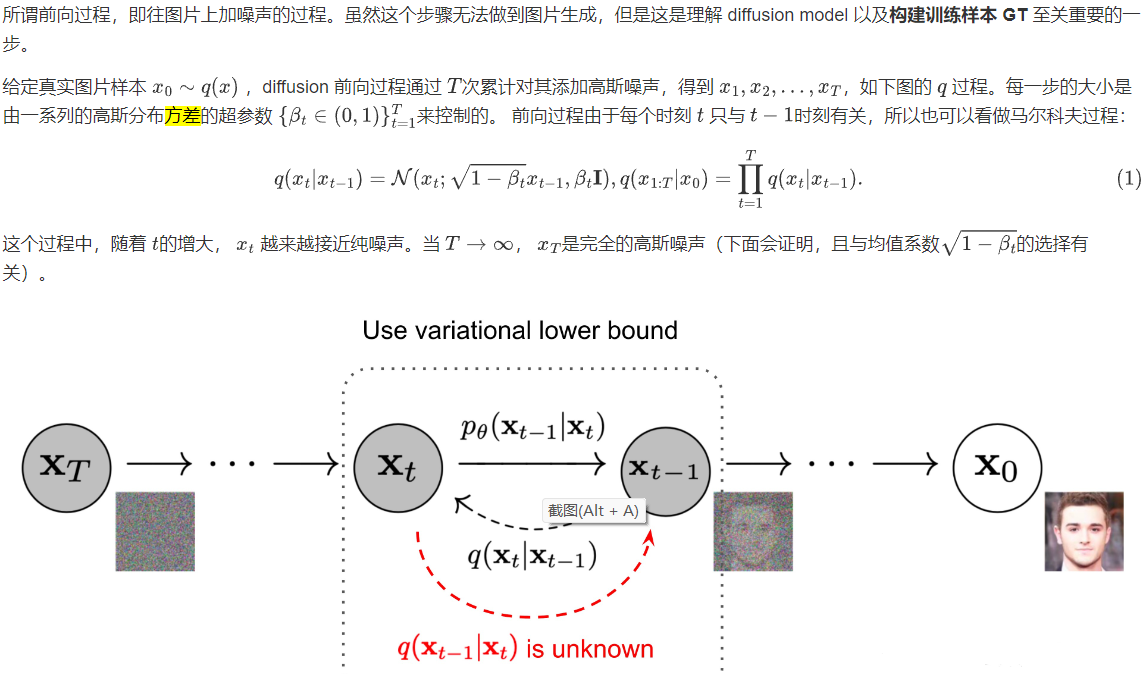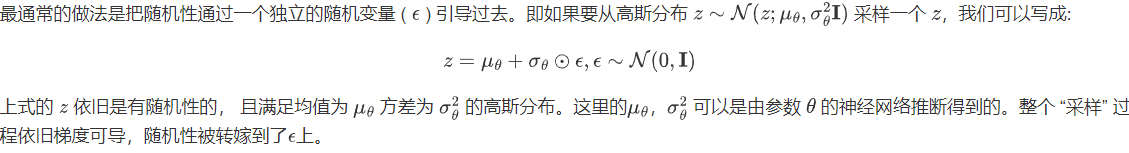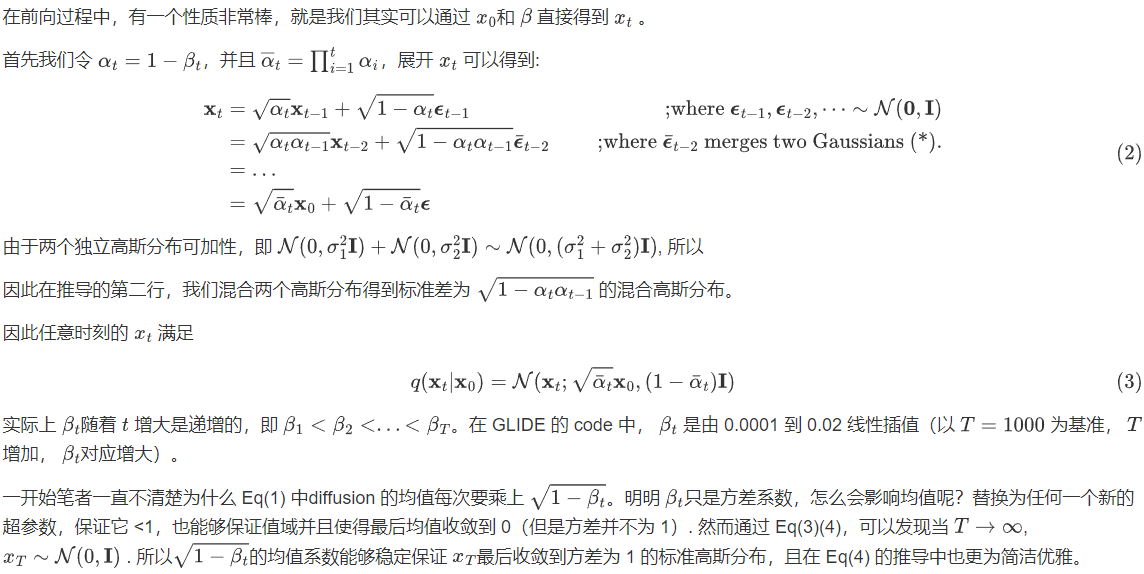## 2.逆扩散过程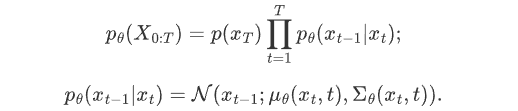## 3.逆扩散条件概率推导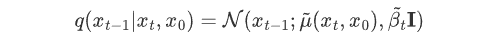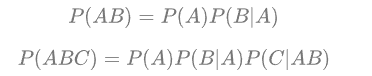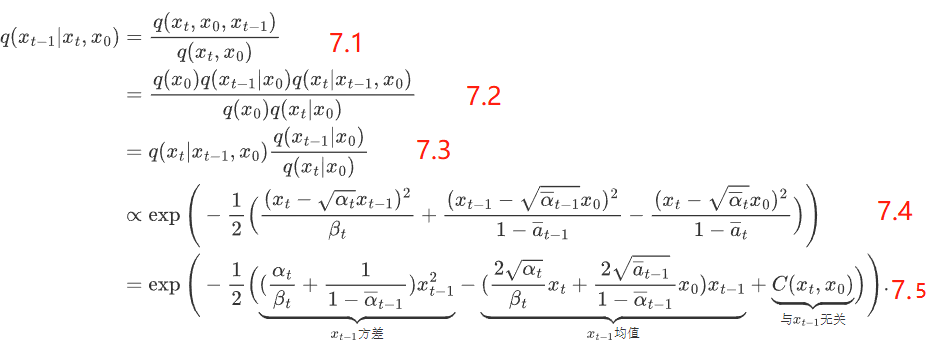7-1带入了贝叶斯公式2；7-2带入乘法公式1，再整理一下就能得到7-3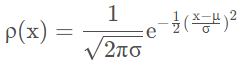，代入得到式 7.4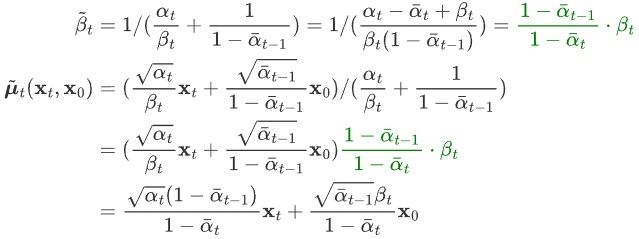## 4.训练损失

Diffusion Models 通过极大似然估计，来找到逆扩散过程中马尔可夫链转换的概率分布，这就是 Diffusion Models 的训练目的。即最大化模型预测分布的对数似然，从Loss下降的角度就是最小化负对数似然：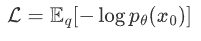KL 散度是一种不对称统计距离度量，用于衡量一个概率分布 P 与另外一个概率分布 Q 的差异程度。连续分布的 KL 散度的数学形式是：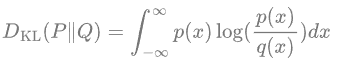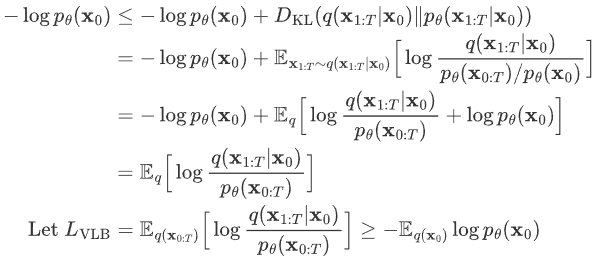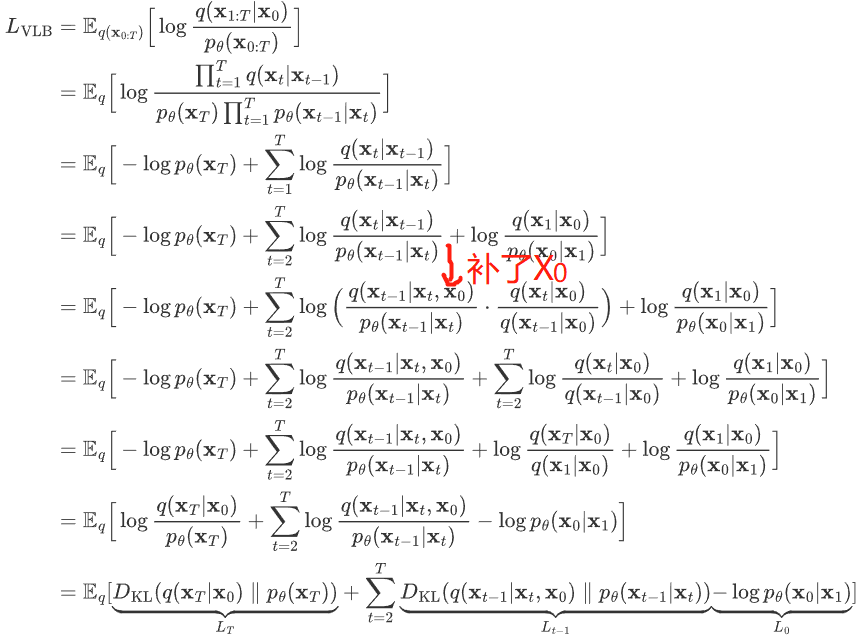1. 第一个分布 q(xt-1|xT,x0,) 我们已经在上一节推导出其解析形式，这是一个高斯分布，其均值和方差为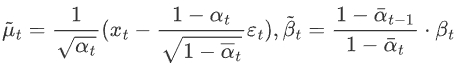2. 第二个分布 pθ(xt-1，xt) 是我们网络期望拟合的目标分布，也是一个高斯分布，均值用网络估计，方差被设置为了一个和 βt 有关的常数。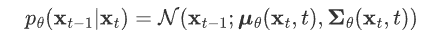如果有两个分布 p,q 都是高斯分布，则他们的KL散度为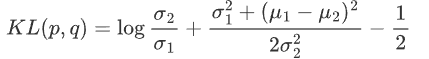然后因为这两个分布的方差全是常数，和优化无关，所以其实优化目标就是两个分布均值的二范数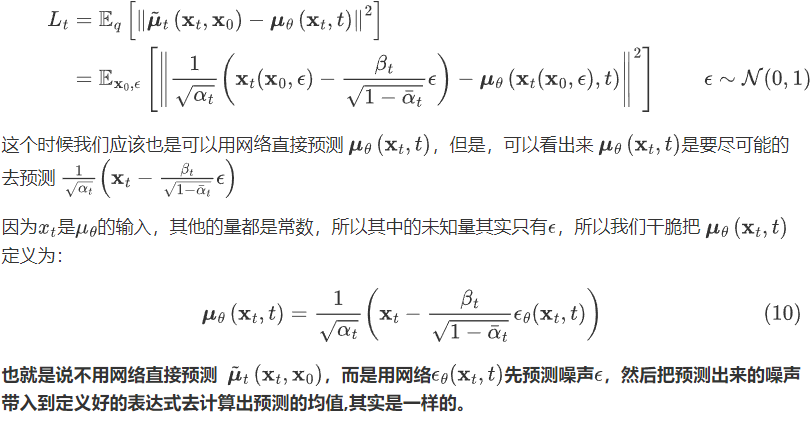把这个公式，带入到 上一公式中得到：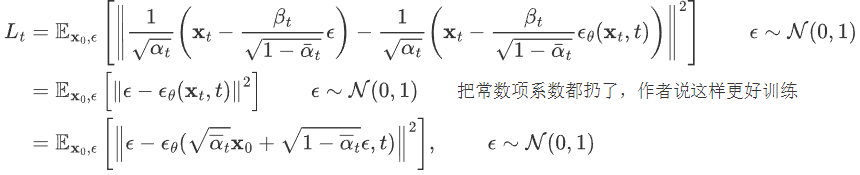经过这样一番推导之后就是个 L2 loss。网络的输入是一张和噪声线性组合的图片，然后要估计出来这个噪声：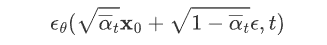# 五、训练、测试伪代码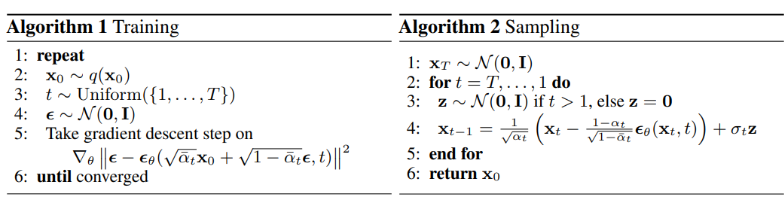## 1. 训练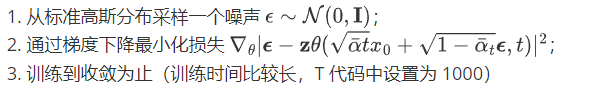## 2.测试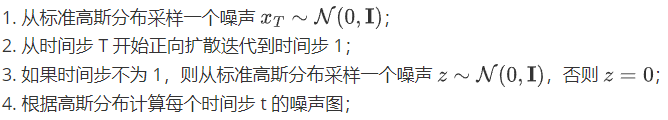# 六、代码解析

github.com/abarankab/DDPM

## 1.train_cifar.py

``````from torchvision import datasets

# 1.定义模型（Unet，后续会展开）
diffusion = script_utils.get_diffusion_from_args(args).to(device)

# 2.迭代器

# 3.从 torchvision 读入数据集
train_dataset = datasets.CIFAR10( root='./cifar_train', train=True,

for iteration in range(1, 80000):
diffusion.train()

if args.use_labels:
loss = diffusion(x, y)
else:
loss = diffusion(x)

``````

``````model = UNet(img_channels=3, base_channels=128)

# 生成 t=1000 对应的 β（0.001~0.02）
if args.schedule == "cosine":
betas = generate_cosine_schedule(args.num_timesteps=1000)
else:
betas = generate_linear_schedule(num_timesteps=1000,
1e-4 * 1000 / args.num_timesteps,
0.02 * 1000 / args.num_timesteps)

diffusion = GaussianDiffusion( model, (32, 32), 3, 10, betas,
ema_decay=0.9999,  ema_update_rate=1, ema_start=2000, loss_type='l2')

return diffusion
``````

time_mlp、init_conv(3,128)、down（12层ResidualBlock）、mid、up（12层Res）组成。time_mlp为 时间步 t 的可学习张量，下面有具体定义代码；
GaussianDiffusion为预设的一系列超参数，如 β、累乘α等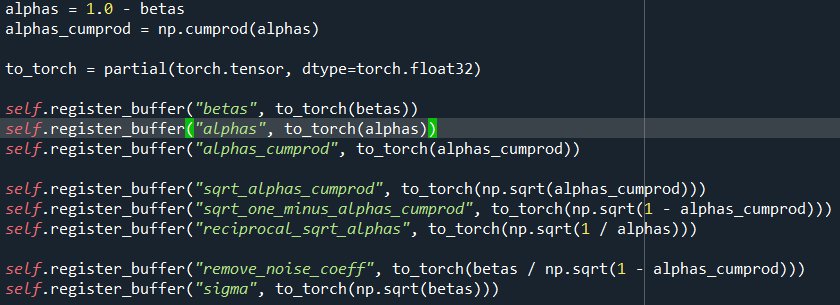``````class PositionalEmbedding(nn.Module):
__doc__ = r"""Computes a positional embedding of timesteps.

Input:
x: tensor of shape (N)
Output:
tensor of shape (N, dim)
Args:
dim (int): embedding dimension
scale (float): linear scale to be applied to timesteps. Default: 1.0
"""

def __init__(self, dim, scale=1.0):
super().__init__()
assert dim % 2 == 0
self.dim = dim
self.scale = scale

def forward(self, x):
device = x.device
half_dim = self.dim // 2
emb = math.log(10000) / half_dim
emb = torch.exp(torch.arange(half_dim, device=device) * -emb)
emb = torch.outer(x * self.scale, emb)
emb = torch.cat((emb.sin(), emb.cos()), dim=-1)
return emb

self.time_mlp = nn.Sequential(
PositionalEmbedding(base_channels=128, time_emb_scale=1.0),
nn.Linear(128, 512),
nn.SiLU(),
nn.Linear(512, 512),
)
``````

``````b, c, h, w = x.shape                    # x:128，3，32，32  y是对应的128个标签

t = torch.randint(0, self.num_timesteps, (b,), device=device)
# 从(0,1000)中随机选128个t

return self.get_losses(x, t, y)
def get_losses(self, x, t, y):
noise = torch.randn_like(x)         # 随机噪声

1.perturbed_x = self.perturb_x(x, t, noise)
# 用x0表示出xt， 下一行是具体操作：
perturbed_x = extract(self.sqrt_alphas_cumprod, t, x.shape) * x +
extract(self.sqrt_one_minus_alphas_cumprod, t, x.shape) * noise

2.estimated_noise = self.model(perturbed_x, t, y)
# 下一行是具体操作：
2.1. time_emb = self.time_mlp(t)         # (128) -> (128,512)
emb = math.log(10000) / half_dim    # 10000/64= 0.143
emb = torch.exp(torch.arange(half_dim, device=device) * -emb)    # (64):[1.0, 0.86, 0.75, ...0.0001]
emb = torch.outer(t * self.scale, emb)        # (128,64) 矩阵乘法
emb = torch.cat((emb.sin(), emb.cos()), dim=-1)   # （128，128）
time_emb = conv2d(emb)              # (128,512)

2.2. for layer in self.downs:
x = layer(x, time_emb, y)    # 将 time_emb 添加到特征中。即：
out += self.time_bias(self.activation(time_emb))[:, :, None, None]
# self.time_bias 是linear（512，128），activation 是silu函数。直接跟特征相加
for layer in self.mid:
x = layer(x, time_emb, y)
for layer in self.ups:
x = layer(x, time_emb, y)

x = self.activation(self.out_norm(x))
x = self.out_conv(x)              # 返回值为噪音（跟输入维度相同）

if self.loss_type == "l1":
loss = (estimated_noise - noise).abs().mean()
elif self.loss_type == "l2":
loss = (estimated_noise - noise).square().mean()

return loss

``````

## 2.sample_images.py（预测过程）

``````x = torch.randn(batch_size, self.img_channels, *self.img_size, device=device)
# 随机采样高斯噪声，作为xt

for t in range(self.num_timesteps - 1, -1, -1):        # T=1000
t_batch = torch.tensor([t], device=device).repeat(batch_size)
x = self.remove_noise(x, t_batch, y, use_ema)      # 得到x(t-1),即：
x = (    (x - extract(self.remove_noise_coeff, t, x.shape) * self.model(x, t, y))
* extract(self.reciprocal_sqrt_alphas, t, x.shape)       )
``````

# 总结

1. Diffusion Model 通过参数化的方式表示为马尔科夫链，这意味着隐变量 x1,…xT 都满足当前时间步 t 只依赖于上一个时间步 t-1，这样对后续计算很有帮助。
2. 马尔科夫链中的转变概率分布 pθ(xt-1|xt) 服从高斯分布，在正向扩散过程当中高斯分布的参数是直接设定的，而逆向过程中的高斯分布参数是通过学习得到的。
3. Diffusion Model 网络模型扩展性和鲁棒性比较强，可以选择输入和输出维度相同的网络模型，例如类似于UNet的架构，保持网络模型的输入和输出 Tensor dims 相等。
4. Diffusion Model 的目的是对输入数据求极大似然函数，实际表现为通过训练来调整模型参数以最小化数据的负对数似然的变分上限
5. 在概率分布转换过程中，因为通过马尔科夫假设，目标函数第4点中的变分上限都可以转变为利用 KL 散度来计算，因此避免了采用蒙特卡洛采样的方式。

(0)